# One-Period Binomial Model

The law of arbitrage dictates that the value of any two assets (or portfolio of assets) whose payoffs are identical in all possible future scenarios at a given time must also be identical today.

Unlike forward commitments that offer symmetric payoffs at a pre-determined price in the future, contingent claims offer asymmetric payoffs. For this reason, their valuation is a challenge. The binomial model can be used to model the payoffs of contingent claims.

## One-Period Binomial Model

The idea behind the binomial model is that at maturity, an asset’s spot price, $$S_0$$, can either increase to $$S_1^u$$ or decrease to $$S_1^d$$. We do not need to know the asset’s future price in advance since it depends on a random variable’s outcome. The asset price movements, from $$S_0$$ to either $$S_1^u$$ or $$S_1^d$$, can be seen as the outcome of a Bernoulli trial.

Let us denote $$q$$ as the probability of an increase in the asset’s price. Because of the presence of only two possibilities, i.e., an increase or a decrease in the asset’s price, we can denote the probability of a decrease in its price as $$1-q$$, such that the two probabilities will add up to 1.

The gross return when the asset price increases will be:

$$R^u=\frac{S_1^u}{S_0}>1$$

When the asset price decreases, the gross return will be:

$$R^d=\frac{S_1^d}{S_0}<1$$

The difference between $$S_1^u$$ (or $$R^uS_o)$$ and $$S_1^d$$ (or $$R^dS_0$$) is the spread of possible future price outcomes.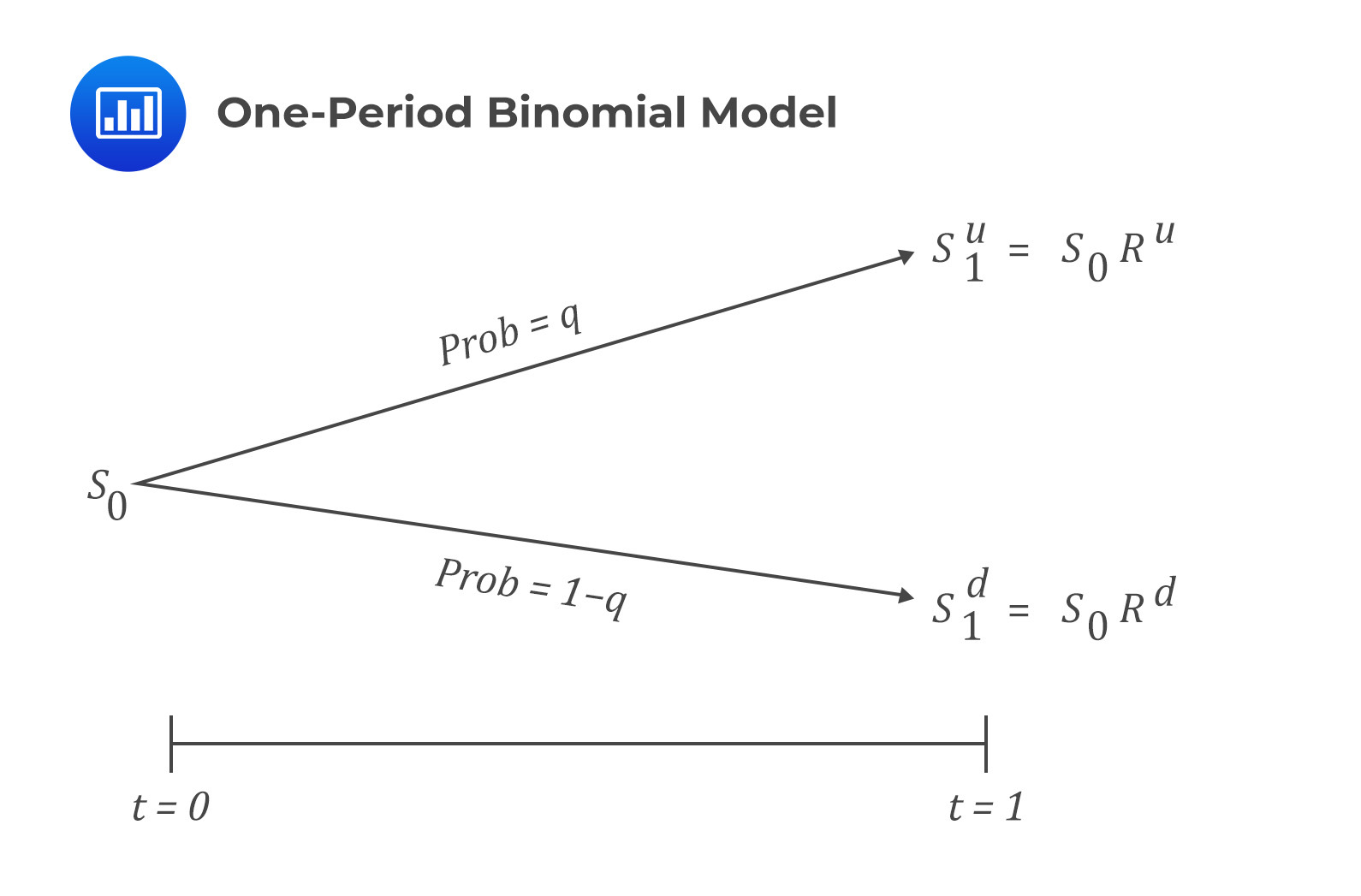### Pricing a European Call Option using a One-Period Binomial Model

Consider a one-year call option with an underlying price of $$S_0$$ and an exercise price of $$X$$. Also, assume that $$S_1^d<X<S_1^u$$ and that the one-period binomial model is equivalent to the time of expiration of one year.

The one-period binomial model gives the underlying asset values in one year, where the option value is defined as a function of the underlying value.

#### At time t=0:

The value of the call option is $$c_0$$. Note that this value is unknown and needs to be calculated.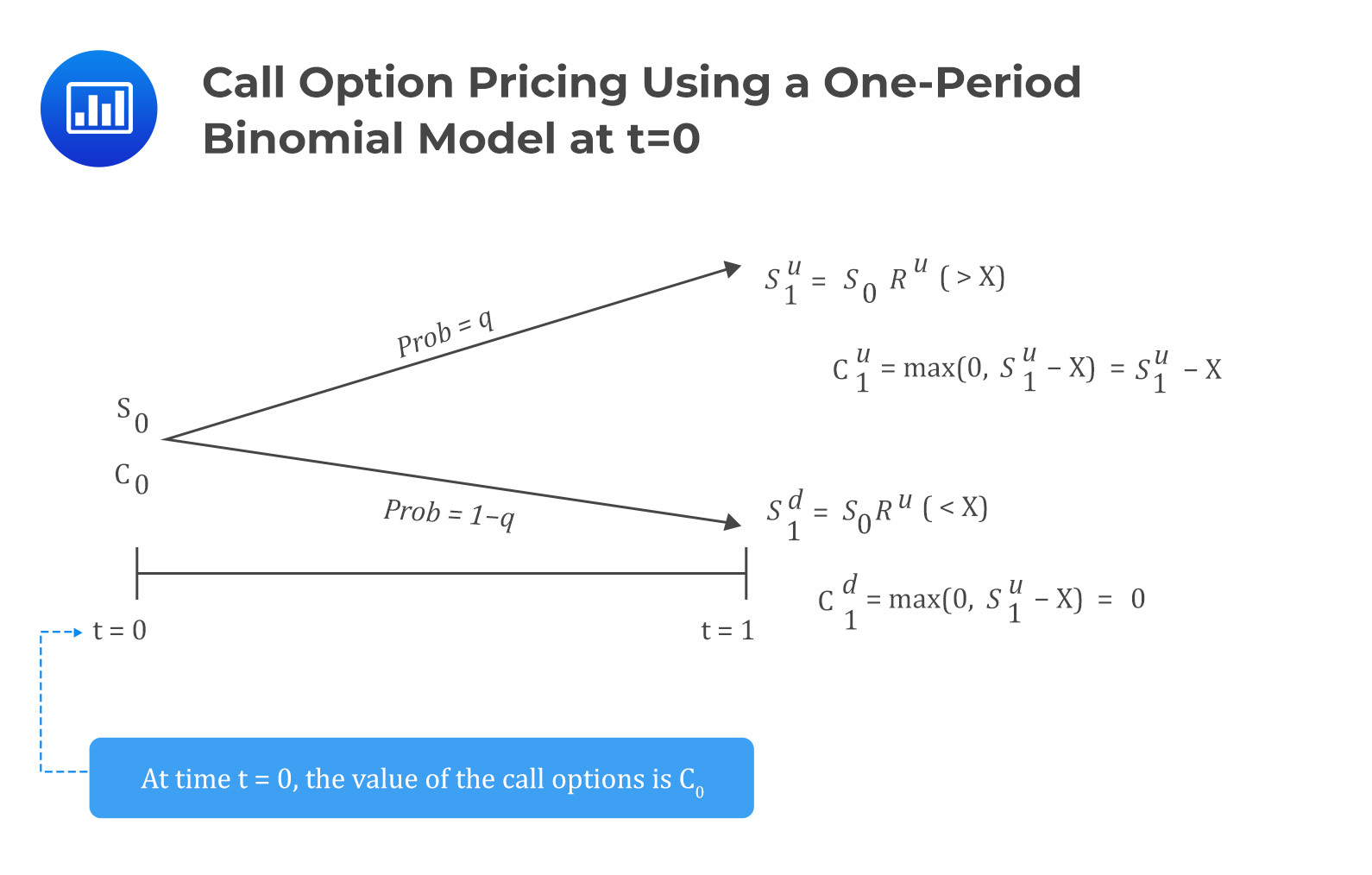#### At time t=1:

After one year, the option expires. At this time, the value of the option will either be $$c_1^u=\max(0,S_1^u-X)$$ if the underlying price rises to $$S_1^u$$ or $$c_1^d=\max(0,S_1^u-X)$$ if the underlying price falls to $$S_1^d$$.

Intuitively, for the up movement, the call option is in the money, and for the down direction, the option is out of the money.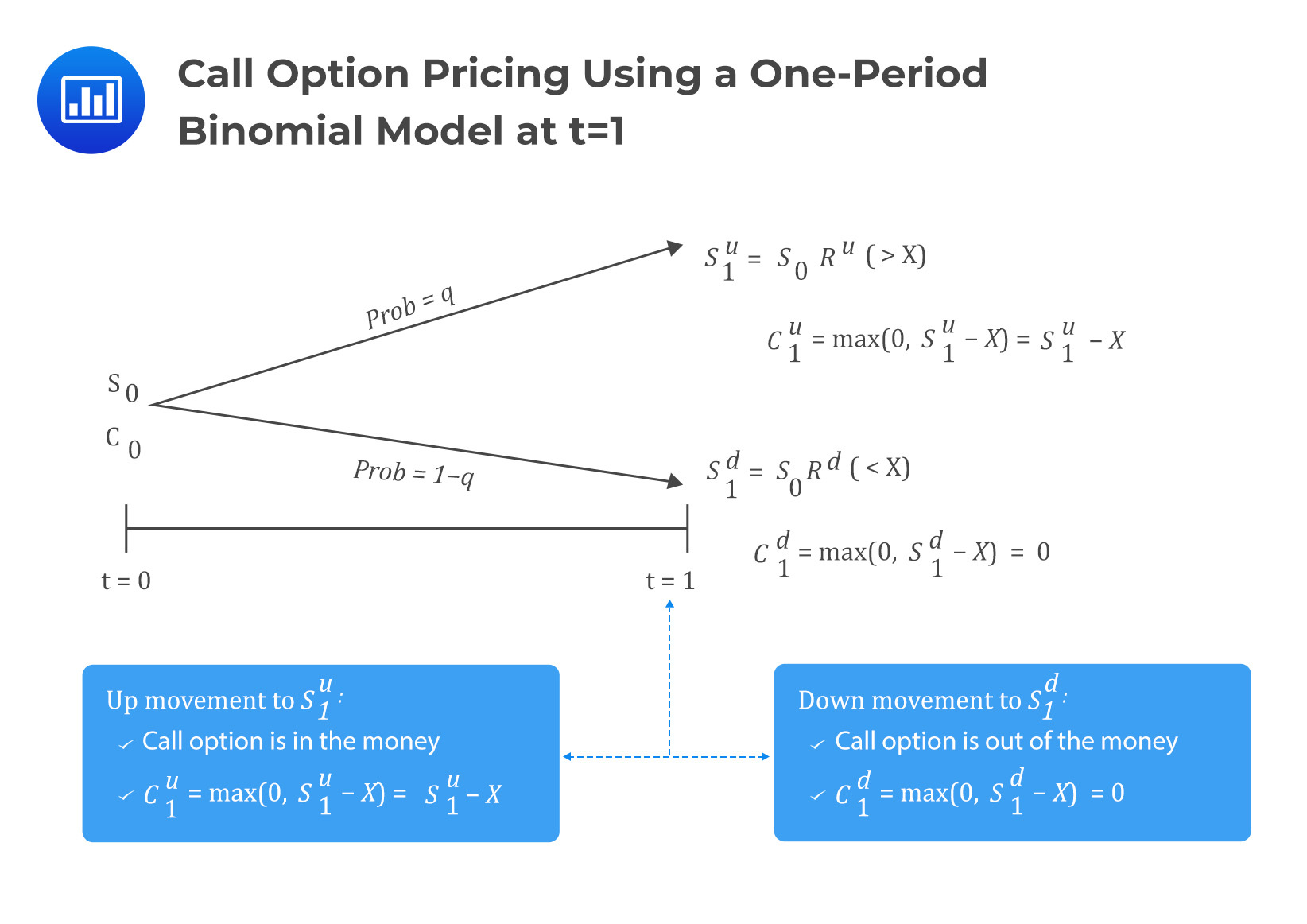#### Determining the Value of c0

The value of $$c_0$$ is determined by applying replication and no-arbitrage pricing. Replication implies that the value of the option and its underlying asset in any future scenario may be used to construct a risk-free portfolio.

With that in mind, assume at time $$t=0$$, we sell a call option for a price of $$c_0$$ and buy $$h$$ units of the underlying asset. Also, let the value of the portfolio be $$V$$ so that its value at $$t=0$$ is:

$$V_0={\rm hS}_0-c_0$$

The value of the portfolio, if the underlying price moves up, is given by:

$$V_1^u={\rm hS}_1^u-c_1^u=h\times R^u\times S_0-\max{\left(0,S_1^u-X\right)}$$

And for the down movement:

$$V_1^d={\rm hS}_1^d-c_1^d=h\times R^d\times S_0-\max{\left(0,S_1^d-X\right)}$$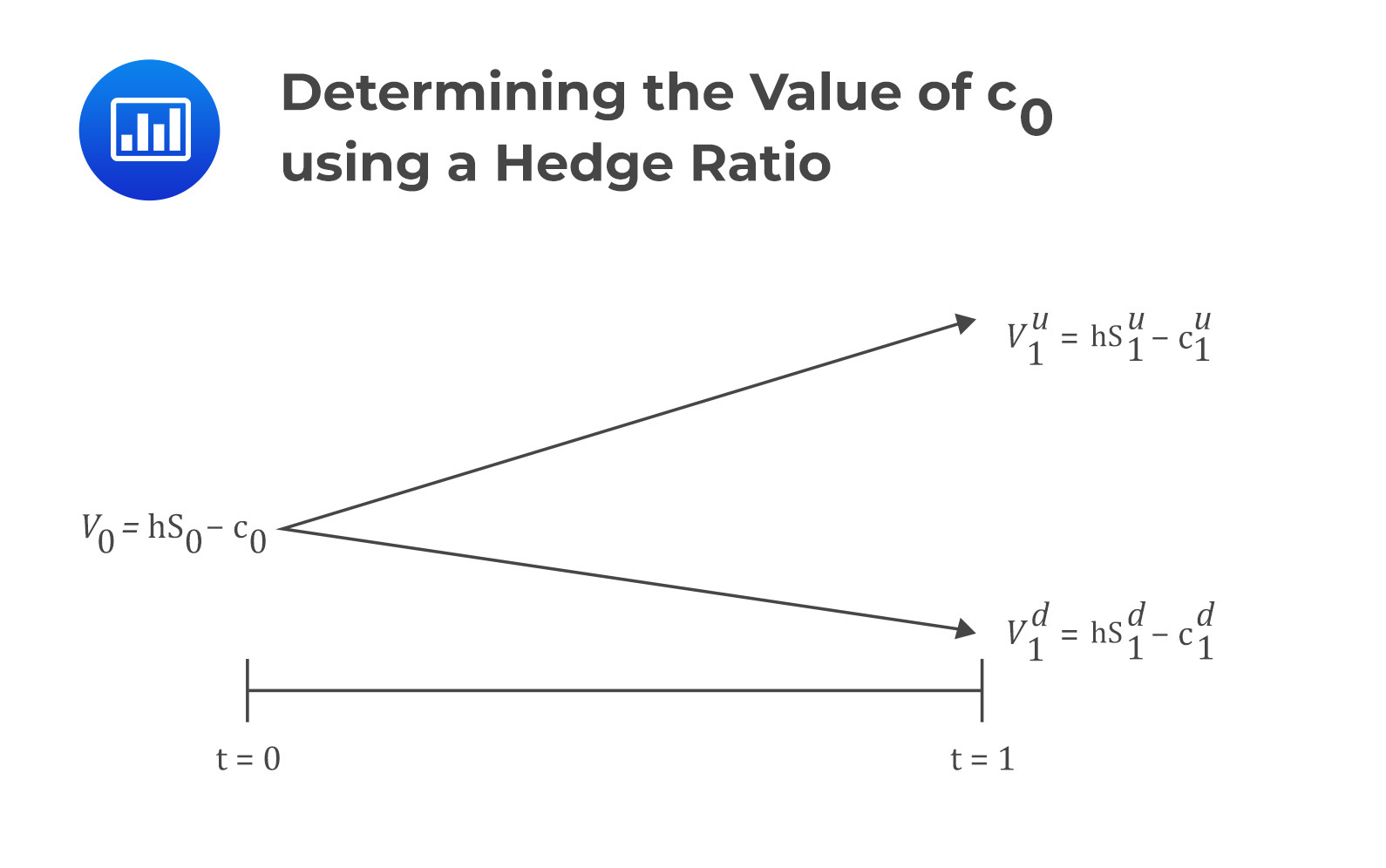Assuming no-arbitrage condition, note that we have established two portfolios with identical payoff profiles at time $$t=1$$. As such, we need to find the value of $$h$$ such that:

$$V_1^u=V_1^d$$

Therefore,

$$\Rightarrow{hS}_1^u-c_1^u={hS}_1^d-c_1^d$$

Making $$h$$ the subject of the formula:

$$h=\frac{c_1^u-c_1^d}{S_1^u-S_1^d}$$

The value $$h$$ is called the hedge ratio. The hedge ratio is a proportion of the underlying that will offset the risk associated with an option.

Since $$V_1^u=V_1^d$$, we can draw two conclusions:

• We can utilize either of the two portfolios to value the option.
• The return $$\frac{V_1^u}{V_0}=\frac{V_1^d}{V_0}=1+r$$.

To avoid arbitrage, the portfolio value at $$t=1$$, ($$V_1={\ V}_1^u=V_1^d$$), must be discounted using a risk-free rate so that:

$$V_0=V_1\left(1+r\right)^{-1}$$

However, recall that $$V_0={hS}_0-c_0$$:

$$\Rightarrow{\rm hS}_0-c_0=V_1\left(1+r\right)^{-1}$$

Making $$c_0$$ the subject of the formula:

$$c_0={\rm hS}_0-V_1\left(1+r\right)^{-1}$$

#### Example: Pricing Call Option Using One Period Binomial Model

A European call option that expires in one year has an exercise price of $70 and an underlying spot price of$60. Use a one-period binomial model to estimate the call option price if the underlying’s spot price is expected to change by 25% in one year. Assume that the risk-free annual rate is 6%.

Solution

Step 1: Determine the Call Option’s Value at Maturity t=1

At maturity, the value can either be $$c_1^u$$ if the price of the underlying goes up or $$c_1^d$$ if the price of the underlying goes down.

The spot price at maturity, if the price goes up by 25%, will be:

$$S_1^u=\frac{125}{100}\times60=75$$

and the gross return will be:

$$R^u=\frac{S_1^u}{S_o}=\frac{75}{60}=1.25$$

If the price goes down by 25%, the spot price at maturity will be:

$$S_1^d=\frac{75}{100}\times60=45$$

and the gross return will be:

$$R_d=\frac{S_1^d}{S_o}=\frac{45}{60}=0.75$$

If the underlying’s price goes up (the call option expires in the money):

$$c_1^u=\max\ \left(0,\ S_1^u-X\right)=\max\ \left(0,\ 75-70\right)=5$$

If the underlying’s price goes down, and the call option expires out of the money:

$$c_1^d=\max\ \left(0,\ S_1^d-X\right)=\max\ \left(0,\ 45-70\right)=0$$

Step 2: Determining h, the Hedge Ratio

The hedge ratio is given by

\begin{align} h&=\frac{c_1^u-c_1^d}{S_1^u-S_1^d}\\&=\frac{5-0}{75-45}=0.167\end{align}

The hedge ratio of 0.167 implies that we either need to buy 0.167 units of the underlying for every call option we sell or sell 6 call options for each underlying asset to equate the portfolio values at maturity ($$t=1$$). Therefore, the portfolio values when the price of the underlying increases and decreases respectively is:

\begin{align}V_1^u&=\left(0.167\times75\right)-5=7.5\\V_1^d&=\left(0.167\times45\right)-0=7.5\end{align}

The portfolio values are the same, implying that either of the portfolios can be used to value the derivative.

Step 4: Determining $$\mathbf{V_0}$$

We can obtain $$V_0$$ by discounting $$V_1$$ at the risk-free discount rate. Remember that $$V_1=V_1^u=V_1^d$$:

$$V_o=7.5(1+{0.05)}^{-1}=7.14$$

Step 5: Determining $$\mathbf{c_0}$$

Recall that:

$$c_0={\rm hS}_0-V_1\left(1+r\right)^{-1}=hS_o-V_0$$

Therefore,

$$c_o=hS_o-V_0=\left(0.167\times60\right)-7.14=2.88$$

Note: The hedging approach can be used to value many derivatives, not just call options, provided the derivative’s value depends on the underlying asset’s value at contract maturity, i.e., $$t=1$$

## Pricing a European Put Option One-period Binomial Model

For put options, the same explanations we gave under the call option apply, albeit with a different replication strategy. Consider the following diagram: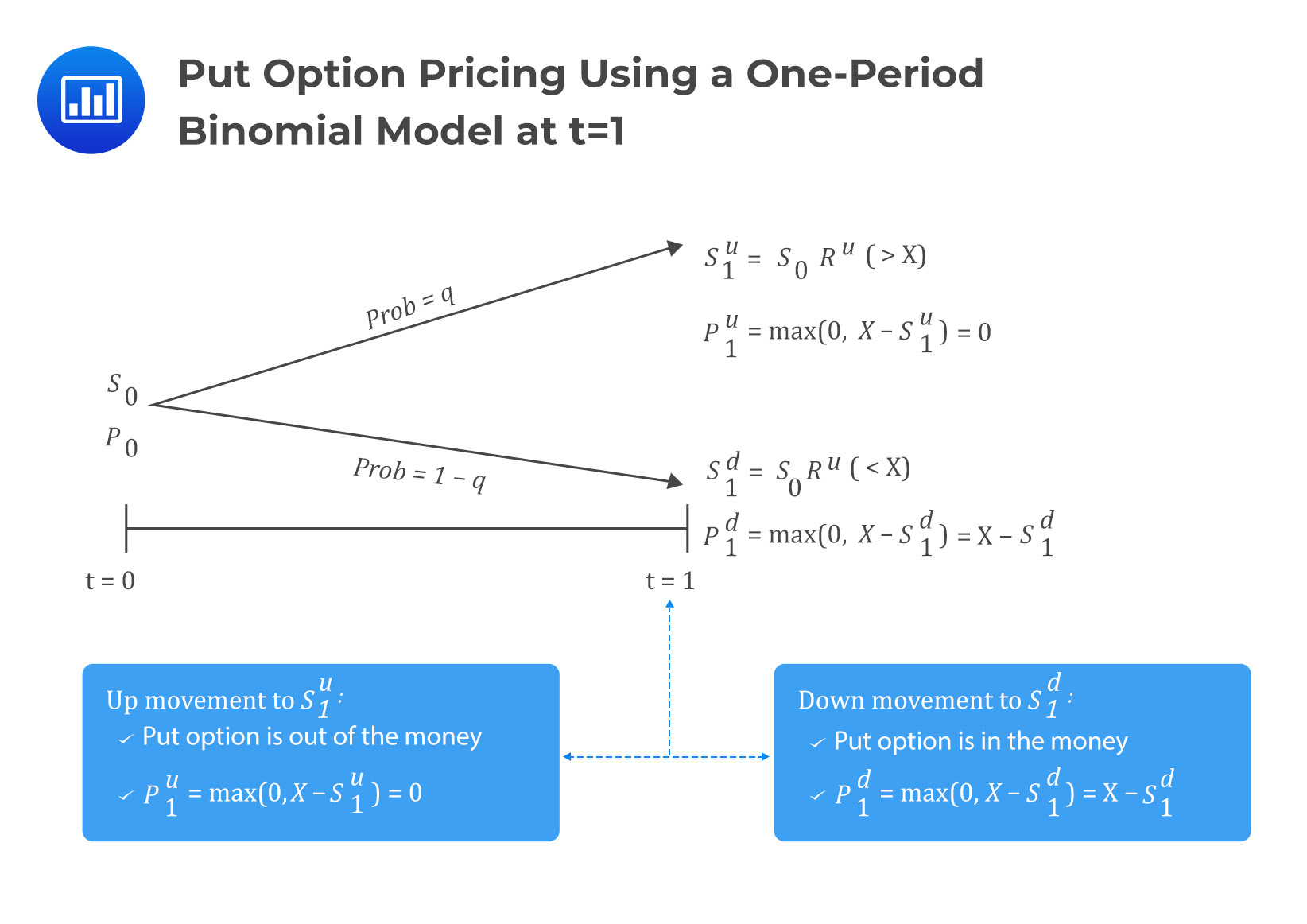Under the put option, the hedge ratio is given by

$$h=\frac{p_1^u-p_1^d}{S_1^d-S_1^u}$$

Note that the formula remains the same as in the call option, except for the change of notations. Replication in pricing put option using a one-period binomial model involves buying the put option and $$h$$ units of the underlying so that:

$$V_0=p_0+hS_0$$

Therefore,

$$p_0=V_0-hS_0$$

## Question

Consider a one-year put option on a non-dividend paying stock with an exercise price of $50. The current stock price is$45. The stock price is expected to go up or down by 20%. Calculate the non-arbitrage price of the put option if the risk-free rate of return is 4%.

A. 0

B. $5.38 C.$14.00

Solution

Consider the following diagram: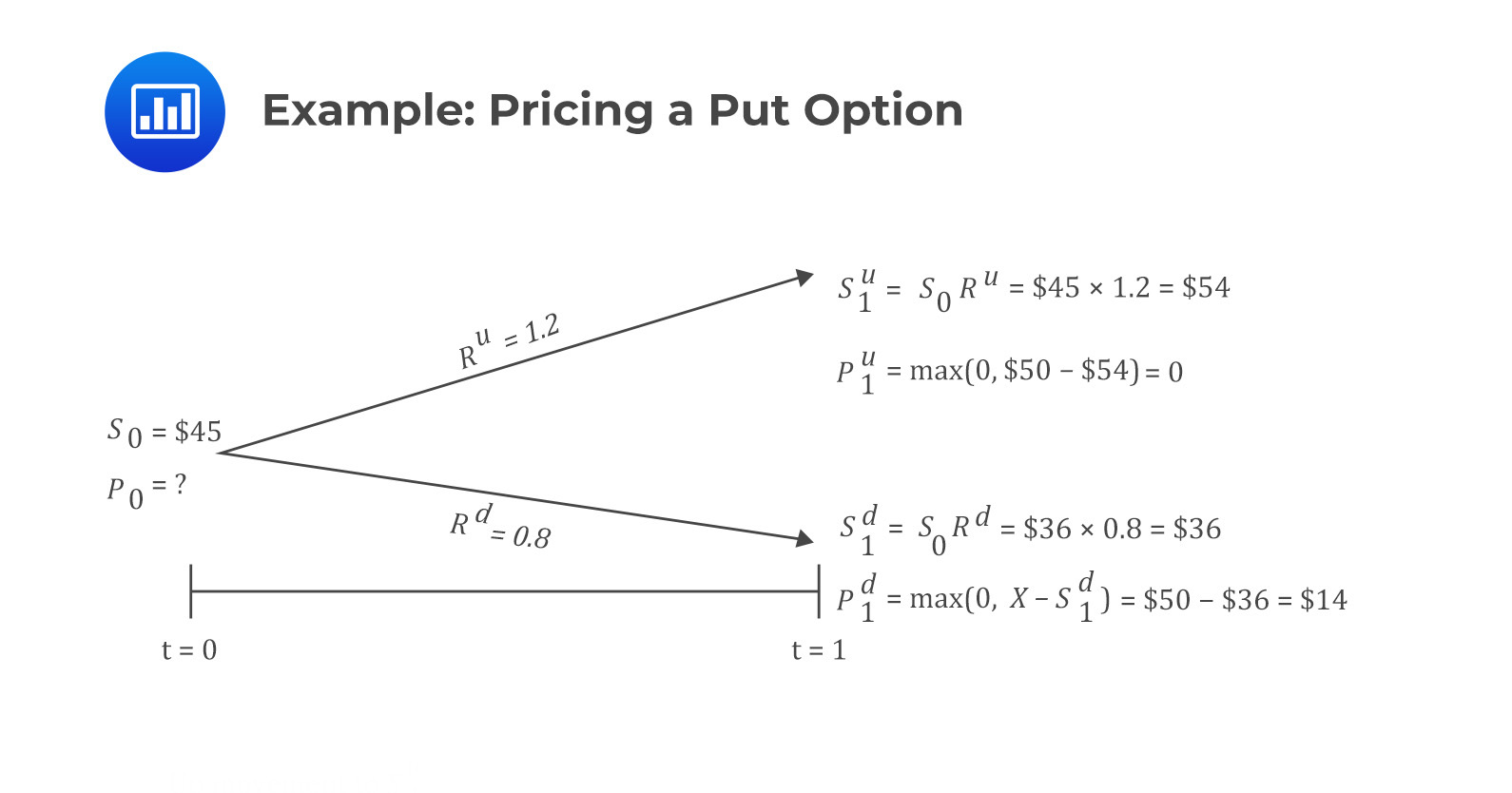From the above results, the hedge ratio is given by:

$$h=\frac{p_1^u-p_1^d}{S_1^u-S_1^d}=\frac{0-14}{54-36}=-0.7778$$

We need to calculate $$V_1={\ V}_1^u=V_1^d$$, which are:

\begin{align}V_1^u&={\rm hS}_1^u+p_1^u=0.7778\times54+0=\42.00\\V_1^d&={\rm hS}_1^d+p_1^d=0.7778\times36+14=\42.00\end{align}

Next, we can either use $$V_1^u$$ or $$V_1^d$$ to calculate the value of $$V_0$$:

$$V_0=V_1\left(1+r\right)^{-1}=42\left(1.04\right)^{-1}=\40.38$$

Therefore, the put option value is:

$$p_0=V_0-{\rm hS}_0=40.38-0.7778\times45=\5.38$$

Shop CFA® Exam Prep

Offered by AnalystPrepLevel I
Level II
Level III
All Three Levels
Featured Shop FRM® Exam PrepFRM Part I
FRM Part II
FRM Part I & Part II
Learn with Us

Subscribe to our newsletter and keep up with the latest and greatest tips for success
Shop Actuarial Exams PrepExam P (Probability)
Exam FM (Financial Mathematics)
Exams P & FM
Shop GMAT® Exam PrepComplete CourseSergio Torrico
2021-07-23
Excelente para el FRM 2 Escribo esta revisión en español para los hispanohablantes, soy de Bolivia, y utilicé AnalystPrep para dudas y consultas sobre mi preparación para el FRM nivel 2 (lo tomé una sola vez y aprobé muy bien), siempre tuve un soporte claro, directo y rápido, el material sale rápido cuando hay cambios en el temario de GARP, y los ejercicios y exámenes son muy útiles para practicar.diana
2021-07-17
So helpful. I have been using the videos to prepare for the CFA Level II exam. The videos signpost the reading contents, explain the concepts and provide additional context for specific concepts. The fun light-hearted analogies are also a welcome break to some very dry content. I usually watch the videos before going into more in-depth reading and they are a good way to avoid being overwhelmed by the sheer volume of content when you look at the readings.Kriti Dhawan
2021-07-16
A great curriculum provider. James sir explains the concept so well that rather than memorising it, you tend to intuitively understand and absorb them. Thank you ! Grateful I saw this at the right time for my CFA prep.nikhil kumar
2021-06-28
Very well explained and gives a great insight about topics in a very short time. Glad to have found Professor Forjan's lectures.Marwan
2021-06-22
Great support throughout the course by the team, did not feel neglectedBenjamin anonymous
2021-05-10
I loved using AnalystPrep for FRM. QBank is huge, videos are great. Would recommend to a friendDaniel Glyn
2021-03-24
I have finished my FRM1 thanks to AnalystPrep. And now using AnalystPrep for my FRM2 preparation. Professor Forjan is brilliant. He gives such good explanations and analogies. And more than anything makes learning fun. A big thank you to Analystprep and Professor Forjan. 5 stars all the way!michael walshe
2021-03-18
Professor James' videos are excellent for understanding the underlying theories behind financial engineering / financial analysis. The AnalystPrep videos were better than any of the others that I searched through on YouTube for providing a clear explanation of some concepts, such as Portfolio theory, CAPM, and Arbitrage Pricing theory. Watching these cleared up many of the unclarities I had in my head. Highly recommended.